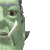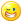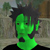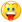## Welcome to the Second Life Forums Archive

These forums are CLOSED. Please visit the new forums HERE

# Make pipes using cylinders and toruses

Lex Neva
wears dorky glasses
Join date: 27 Nov 2004
Posts: 1,361
02-05-2006 12:06
My friend was building a system of pipes using cylinders and torus pieces. I'm not sure what came over me, but I got inspired to see if I could work out the math to make a script that would make her job much easier. A day later, I'm happy to release the PipeMaker to the forums. I hope everyone gets a lot of use out of it.

The PipeMaker lets you create long, continuous lines of piping using cylinders and torus pieces that line up perfectly, end to end. You can continue for as long as you want, and you can adjust several parameters of the pipes. This version lets you change the length of straight segments, and the radius, arc, and tilt of curved segments, which should be enough to create some really nifty industrial pipework sculptures in a flash.

Right now, it's good enough for what I originally wrote it for. If someone else wants to do some more serious math, I could see the following features being good additions:

* Make it use boxes and toruses, or cut boxes and rings. This'd require some kinky math involving twist settings.
* Make it allow tapering. More kinky math.
* Make a random pipe generation mode. You really ought to make sure the thing has a hard limit so it doesn't make pipes until the sim is full ;)
* Make it drag along the texture used on the first pipe, not just the hollow and size settings.
* Allow backspacing.

Anyway, here's the script as it stands. There's a little setup required, or you can just pick up a copy at Eldora 96, 168, 106 (this may go away soon). I've also got a few pipe doodles laid out there, including my name written in cursive. I'd post a screenshot, but attachments are disabled right now.

Here's the script:

CODE
`// PipeMaker by Lex Neva//// Pipemaker helps you easily create continuous lengths of pipe using cylinders and torus segments.  The simple dialog-driven// interface always lines pieces up perfectly to give you one long continuous pipe.  It's useful for creating industrial // complicated pipe messes, weird curlicues, and, of course, for writing your name in prims in the air.//// This script is released under the GNU Public License (http://www.gnu.org for a copy).  In short, this means you may copy,// redistribute, and modify this script to your heart's content, so long as my name stays in it and your new version is also// freely redistributable.  For more details, read the full license.  If you really must provide me with some kind of compensation// for my work, feel free to heap praises upon me in the forums, rate me, and/or send me lindens.  You can also check out my // store in Eldora.//// Okay, now that's out of the way.  Here's how to use this thing.//// If you've managed to pick up a copy of this script in the wild, you'll have to build the object.  Fortunately, that's easy.//// 1. Build a default cylinder, name it PipeMaker (the name's important)// 2. Place this script in the PipeMaker cylinder.// 3. Take a copy.  (leave one rezzed)// 4. Find the copy in your inventory, and place it in the rezzed one.// 5. Take the whole mess into your inventory.// 6. Rez a copy and get started!//// The above process is necessary because the pipemaker uses a safe form of self-replication.//// Now, how to actually make pipes.//// You should be able to just rez the PipeMaker object and go to it.  A dialog will pop up, and a new pipe piece will stick itself// onto the end of the first one.  This new piece (the red one) is the one you're currently working on.  Poke a few buttons.  // Commands like "length+" will make the length of a straight piece longer.  The more pluses, the longer it'll get.  Minuses will// shrink it instead.//// Click the "curve" button if you want to turn a corner instead.  The radius commands will change how sharp the curve is: bigger// radius means a more gradual curve.  The arc commands will determine how much of a circle the curved piece uses.  90 means to// turn a right angle, 180 means to do a "U" bend, etc.  360 probably won't be useful, but the option IS there.  Tilt will // make the curved piece rotate up or down.  Just play with it, you'll get it.//// There are only so many buttons allowable in a script dialog, so I wasn't able to include all of the commands I wanted to.  You// can still do things like "radius+++" if the radius+ button isn't going fast enough: just type /1 radius+++.  You can use up to// three pluses or minuses.//// When you're done, just delete the extra red piece.  I didn't have enough room for a "finish" button.//// If you want to have a hollow pipe, rez the first PipeMaker, and change its hollow value.  Tweak the length so that the red// pipe updates.  From then on, the hollow will carry through.  You could, if you want, change the hollow at any time, and// the setting will carry on to pieces after that one.  If you want to change the thickness of the pipe, just change the// X and Y size of the starting cylinder.  I recommend that the X and Y settings should be the same.  I was too lazy to make// it work with oval pipes.  You can change the size any time, but I don't recommend messing with curved pieces, because getting// everything to match up nicely is difficult.//// The rest is for advanced users.  You can skip this stuff.//// Why isn't there finer control for arc and tilt?  Why does radius change in such weird increments?//// The simple answer is that the object properties this script uses, such as hole size and cut, only allow two decimal places of // precision.  If I let you use any arc value, SL will "round" to the nearest 18 or so degrees, and that'll mean the next pipe// doesn't join on perfectly.  Radius is even more complicated.  You can set the radius of a torus to a fairly fine degree of// precision, but the real limiting factor is the hole size.  If the hole size that would be needed for a certain radius // of curve doesn't line up on an even 0.01, the pipe's cross-section won't match up.  When you use the radius+ and radius- // buttons, I actually decrement and increment the hole size, and figure out the torus's radius from that, which guarantees that// everything lines up beautifully.  Incidentally, I also change the tilt value in increments of 15 degrees, because that way// the vertices of the cylinders and tori always line up nicely.//// That said, you can override these limits manually if you really want to.  I figure if you want to do this, you probably have// a good reason.  Just utter these commands on channel 1 (by prefixing them with "/1")://// radius 2.5// tilt 35.0// arc 90.0// length 5.0// radius+++// (any other command on the buttons)// reset (use only if the red prim fails to rez or is accidentally deleted)//// Just be careful, especially with radius.  If you don't watch it, you can find that things aren't lining up nicely... it's just // due to the limits of primitive properties.  The dialog optiosn should give you a fair degree of freedom.// float torus_radius;float torus_rot;float torus_arc;float cylinder_length;integer type;integer channel;integer listenHandle;vector offsetRot(vector initial_position, vector center_position, rotation rot_amount){        vector offset = initial_position - center_position;            vector final_position;                        //The following line calculates the new coordinates based on     //the rotation & offset    final_position = offset * rot_amount;        //Since the rotation is calculated in terms of our offset, we need to add    //our original center_position back in - to get the final coordinates.    final_position += center_position;        return final_position;}makeTorus() {    //llSay(0,"Making torus part with radius " + (string)torus_radius + " rotation " + (string)torus_rot + " arc " + (string)torus_arc);        vector myScale = llGetScale();    rotation torus_rotation;    vector torus_position;    vector torus_scale;    vector torus_cut;    vector torus_hole_size;    list params = llGetPrimitiveParams([PRIM_TYPE]);    float hollow = llList2Float(params,3);    if (llList2Integer(params,0) == PRIM_TYPE_CYLINDER) {            torus_rotation = llGetRot();        torus_position = llGetPos() + llRot2Up(torus_rotation) * myScale.z * 0.5 - llRot2Left(torus_rotation) * (torus_radius - myScale.x/2.0);                vector face_center = llGetPos() + llRot2Up(torus_rotation) * myScale.z * 0.5;        rotation rot = llAxisAngle2Rot(llRot2Up(torus_rotation), DEG_TO_RAD * torus_rot);                torus_position = offsetRot(torus_position, face_center, rot);        torus_rotation = torus_rotation * rot;        torus_scale = <myScale.x, torus_radius*2.0, torus_radius*2.0>;        torus_hole_size = <1.0,myScale.y/(2*torus_radius),0.0>;            } else {        vector cut = llList2Vector(params,2);        vector hole_size = llList2Vector(params,5);        float d = hole_size.y * myScale.y;                torus_rotation = llGetRot();        torus_position = llGetPos();                torus_rotation *= llAxisAngle2Rot(llRot2Fwd(torus_rotation),cut.y*TWO_PI);        torus_position += llRot2Left(torus_rotation) * (myScale.z/2.0 - torus_radius);                vector face_center = torus_position + llRot2Left(torus_rotation) * (torus_radius - d/2.0);        rotation rot = llAxisAngle2Rot(llRot2Up(torus_rotation), DEG_TO_RAD * torus_rot);                torus_position = offsetRot(torus_position, face_center, rot);        torus_rotation = torus_rotation * rot;                  torus_scale = <d, torus_radius*2.0, torus_radius*2.0>;         torus_hole_size = <1.0,d/(2*torus_radius),0.0>;                  }            torus_cut = <0.0, torus_arc/360.0, 0.0>;        llSay(channel,"TORUS," + (string)torus_position + "," + (string)torus_rotation + "," + (string)torus_scale + "," + (string)torus_cut + "," + (string)hollow + "," + (string)torus_hole_size);}makeCylinder() {    //llSay(0,"Making cylinder with length " + (string)cylinder_length);    vector myScale = llGetScale();    rotation cylinder_rotation;    vector cylinder_position;    vector cylinder_scale;    float d;    list params = llGetPrimitiveParams([PRIM_TYPE]);    float hollow = llList2Float(params,3);    if (llList2Integer(params,0) == PRIM_TYPE_TORUS) {        vector cut = llList2Vector(params,2);        vector hole_size = llList2Vector(params,5);        d = hole_size.y * myScale.y;                    cylinder_rotation = llGetRot();        rotation rot = llAxisAngle2Rot(llRot2Fwd(cylinder_rotation),cut.y*TWO_PI);                cylinder_rotation *= rot;                cylinder_position = llRot2Left(llGetRot()) * ((myScale.y - d)/2.0);        cylinder_position *= rot;        cylinder_position += llGetPos();        cylinder_position += llRot2Up(cylinder_rotation) * (cylinder_length/2.0);    } else {        d = myScale.y;        cylinder_position = llGetPos();        cylinder_rotation = llGetRot();        cylinder_position += llRot2Up(cylinder_rotation) * ((myScale.z + cylinder_length)/2.0);    }        cylinder_scale = <d,d,cylinder_length>;            llSay(channel,"CYLINDER," + (string)cylinder_position + "," + (string)cylinder_rotation + "," + (string)cylinder_scale + "," + (string)hollow);}update() {    if (type == PRIM_TYPE_CYLINDER)        makeCylinder();    else            makeTorus();}rezNext() {    channel = 10000 + llFloor(llFrand(1000000));        //llOwnerSay("Channel = " + (string)channel);        llRezObject("PipeMaker",llGetPos(), ZERO_VECTOR, ZERO_ROTATION, channel);    llSleep(1.0);}sendDialog() {        if (type == PRIM_TYPE_CYLINDER) {        llDialog(llGetOwner(),"Straight piece\n\nlength = " + (string)cylinder_length + " meters",["length+","length++","length+++","length-","length--","length---","curve","next"],1);    } else {        llDialog(llGetOwner(),"Curve piece\n\nradius = " + (string)torus_radius + " meters\narc = " + (string)torus_arc + " degrees\ntilt = " + (string)torus_rot + " degrees",["tilt+","tilt++","next","tilt-","tilt--","straight","radius+","arc+","arc++","radius-","arc-","arc--"],1);    }}float getDiameter() {    vector scale = llGetScale();    return scale.x;}init() {    float d = getDiameter();        if (d > 2.5) {        torus_radius = d;    } else {        torus_radius = d*2.0;    }        torus_rot = 0.0;    torus_arc = 90.0;    cylinder_length = 1.0;    type=PRIM_TYPE_CYLINDER;}            default{       on_rez(integer param) {        if (param) {            llListen(param,"","","");            llSetColor(<1.0,0.0,0.0>,ALL_SIDES);        } else {            llSetColor(<1,1,1>,ALL_SIDES);                        state configure;        }    }                listen(integer c, string name, key id, string message) {        list params = llCSV2List(message);        list primParams;        if (llList2String(params,0) == "TORUS") {            primParams =           [PRIM_POSITION, (vector)llList2String(params,1),                                    PRIM_ROTATION, (rotation)llList2String(params,2),                                    PRIM_SIZE, (vector)llList2String(params,3),                                    PRIM_TYPE,                                     PRIM_TYPE_TORUS,                    // type                                    PRIM_HOLE_DEFAULT,                  // hole type                                    (vector)llList2String(params,4),    // cut                                    (float)llList2String(params,5),   // hollow                                    <0.0,0.0,0.0>,                      // twist                                    (vector)llList2String(params,6),    // hole size                                    <0.0,0.0,0.0>,                      // top shear                                    <0.0,1.0,0.0>,                      // advanced cut                                    <0.0,0.0,0.0>,                      // taper                                    1.0,                                // revolutions                                    0.0,                                // radius offset                                    0.0                                 // skew                                   ];        } else if (llList2String(params,0) == "CYLINDER") {            primParams =           [PRIM_POSITION, (vector)llList2String(params,1),                                    PRIM_ROTATION, (rotation)llList2String(params,2),                                    PRIM_SIZE, (vector)llList2String(params,3),                                    PRIM_TYPE,                                     PRIM_TYPE_CYLINDER,                 // type                                    PRIM_HOLE_DEFAULT,                  // hole type                                    <0.0,1.0,0.0>,                      // cut                                    (float)llList2String(params,4),   // hollow                                    <0.0,0.0,0.0>,                      // twist                                    <1.0, 1.0, 0.0>,                    // top size                                    <0.0,0.0,0.0>                       // top shear                                   ];        } else if (llList2String(params,0) == "DONE") {            llSetColor(<1.0,1.0,1.0>,ALL_SIDES);            state configure;        }                llSetPrimitiveParams(primParams);                                        }            }state configure {    state_entry() {        listenHandle = llListen(1,"",llGetOwner(),"");                init();                sendDialog();                        rezNext();        update();    }        on_rez(integer param) {        llListenRemove(listenHandle);        listenHandle = llListen(1,"",llGetOwner(),"");                llSetColor(<1,1,1>,ALL_SIDES);                init();                sendDialog();                rezNext();        update();    }                changed(integer change) {        if (change & CHANGED_SHAPE) {            // hollow            update();        } else if (change & CHANGED_SCALE) {            float d = getDiameter();                        // must make sure the hole size will be nice and prim size will be under 10.0            if (d > 2.5)                 torus_radius = d;            else                torus_radius = d * 2.0;             update();        }    }        moving_end() {        update();    }        listen(integer c, string name, key id, string message) {        list parts = llParseString2List(message,[" "],[]);        string command = llList2String(parts,0);                // start with chat-only commands, don't resend dialog (it gets spammy)        if (command == "radius") {            torus_radius = (float)llList2String(parts,1);        } else if (command == "rot" || command == "tilt") {            torus_rot = (float)llList2String(parts,1);        } else if (command == "arc") {            torus_arc = (float)llList2String(parts,1);        } else if (command == "length") {            cylinder_length = (float)llList2String(parts,1);        } else if (command == "done" || command == "next") {            llSay(channel,"DONE");                        // now commit suicide            llRemoveInventory(llGetScriptName());            state default; // and just in case, stop doing anything until the script's removed.        } else {             // dialog-driven commands follow, this big else block is so I can send a dialog only for dialog commands                    if (command == "curve") {                type = PRIM_TYPE_TORUS;            } else if (command == "straight") {                type = PRIM_TYPE_CYLINDER;            } else if (llGetSubString(command,0,5) == "length") {                integer strength = llStringLength(command) - 6;                float change = llList2Float([0.1,0.5,1.0],strength - 1);                                if (llGetSubString(command,6,6) == "-")                    change *= -1;                                cylinder_length += change;                                if (cylinder_length < 0.01)                    cylinder_length = 0.01;                if (cylinder_length > 10.0)                    cylinder_length = 10.0;            } else if (llGetSubString(command,0,5) == "radius") {                integer strength = llStringLength(command) - 6;                float change = llList2Float([0.01,0.05,0.1], strength - 1);                                if (llGetSubString(command,6,6) == "+")                    change *= -1;                                float d = getDiameter();                float hole_size = d/(torus_radius * 2.0);                                hole_size += change;                                 if (hole_size < 0.05) {                    //llOwnerSay("clamped to 0.05");                    hole_size = 0.05;                } if (hole_size > 0.5) {                    //llOwnerSay("clamped to 0.5");                    hole_size = 0.5;                }                                float new_radius = d/(hole_size * 2.0);                                if (new_radius <= 5.0) {                    torus_radius = new_radius;                }            } else if (llGetSubString(command,0,2) == "arc") {                integer strength = llStringLength(command) - 3;                float change = llList2Float([0.05,0.1,0.2], strength - 1);                                if (llGetSubString(command,3,3) == "-")                    change *= -1;                                                float cut = torus_arc / 360.0;                                cut += change;                                if (cut > 1.0)                    cut = 1.0;                if (cut < 0.05)                    cut = 0.05;                                    torus_arc = cut * 360.0;            } else if (llGetSubString(command,0,3) == "tilt") {                integer strength = llStringLength(command) - 4;                float change = llList2Float([15.0,45.0,90.0], strength - 1);                                if (llGetSubString(command,4,4) == "-")                    change *= -1;                                                torus_rot += change; // boundaries wrap            } else if (command == "reset") {                init();                rezNext();            } else {                return; // invalid command            }                        sendDialog();        }                // in any case, if we get here, it's time to update the next segment's properties        update();    }        touch_start(integer num) {        sendDialog();    }        object_rez(key id) {        llGiveInventory(id, "PipeMaker");    }}`

Some of the word-wrapping the forums did is kinda nasty... you may just want to go take a copy of the one in Eldora, or IM me for one.
Nada Epoch
The LibrarianJoin date: 4 Nov 2002
Posts: 1,423
Discussion thread
02-05-2006 19:39
/54/cc/86676/1.html
_____________________
i've got nothing.Lex Neva
wears dorky glasses
Join date: 27 Nov 2004
Posts: 1,361
02-06-2006 09:23
I've updated the script above to the newest version, which has some bugfixes for resizing and huge pipes. You can get the newest version at YadNi's now.
MeLight Korvin
Im on da UseJoin date: 4 Jun 2005
Posts: 99
03-14-2006 09:16
Totally amazing, lots of fun!! Have no idea what to use it for, coz i dont build, but im sure heavy builders will find it ultra handy_____________________
Boobs are remote controls for the male brain. Lemmie push some buttons!!!